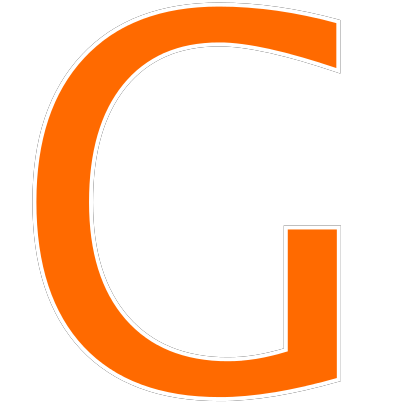# Muscle Freak Maths Puzzle Answer (SOLVED)

Muscle Freak Puzzle Answer, Muscle Freak Maths Puzzle is the new puzzle trending on Facebook, Twitter & WhatsApp. In this Image Puzzle you have to find out the value of weight + man x mask. It is a simple mathematics based riddle in which we have to use BODMAS rule to find out the correct answer. Most people fail to find the solution to this puzzle and end with the wrong answer.

So no need to worry, today in this post we are going to solve this Muscle Freak Maths Puzzle and provide the correct Muscle Freak Puzzle answer. Just scroll down to get the answer and solution for the puzzle.

The Muscle Freak Maths Puzzle Answer is 44

## Muscle Freak Maths Puzzle Solution

Let’s find the Muscle Freak Puzzle solution.

First, we have to find the value of each man. So Man = 15/3 = 5

Now the value of Mask = 9/3 = 3

Whereas Dumble = 18/3 = 6

So 1 Dumble Plate = 6/6=1

Finally, now we have the value of Man, Mask, Dumble, and Dumble Plate. So we can find a solution.

The final equation is:-

Dumbles 2 pair with 4 plates each + Man with mask and dumble with 4 plates x one mask

= (4+4) + (5+3+4) x 3

= 8 + 12 x 3 = 44

So the correct answer to the Muscle Freak Maths Puzzle is 44